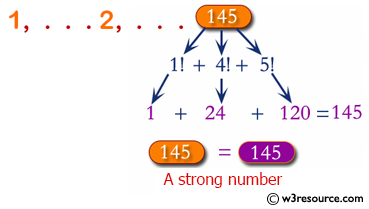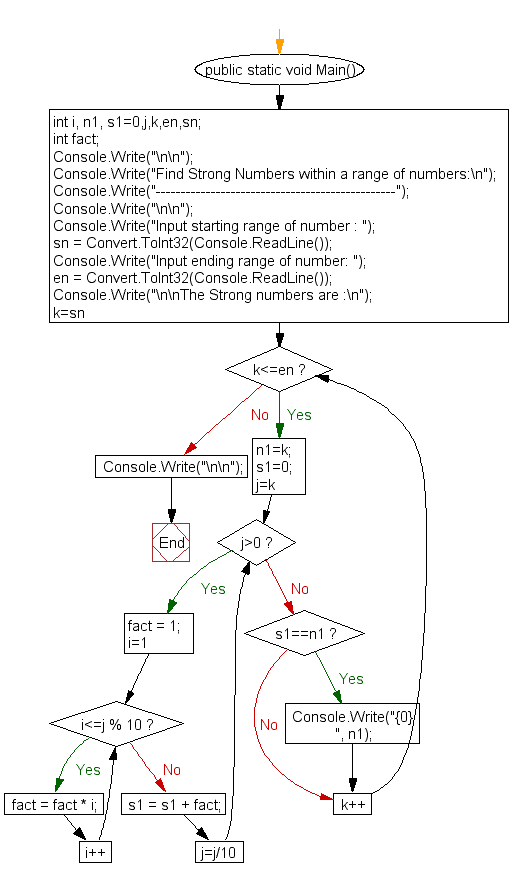﻿ C# - Find Strong Numbers within a range of numbers# C# Sharp Exercises: Find Strong Numbers within a range of numbers

## C# Sharp For Loop: Exercise-48 with Solution

Write a C# Sharp program to find Strong Numbers within a range of numbers.

Pictorial Presentation:Sample Solution:

C# Sharp Code:

``````using System;
public class Exercise48
{
public static void Main()
{
int i, n1, s1=0,j,k,en,sn;
int fact;

Console.Write("\n\n");
Console.Write("Find Strong Numbers within a range of numbers:\n");
Console.Write("------------------------------------------------");
Console.Write("\n\n");

/* If sum of factorial of digits is equal to original number then it is Strong number */

Console.Write("Input starting range of number : ");
Console.Write("Input ending range of number: ");
Console.Write("\n\nThe Strong numbers are :\n");

for(k=sn;k<=en;k++)
{
n1=k;
s1=0;

for(j=k;j>0;j=j/10)
{

fact = 1;
for(i=1; i<=j % 10; i++)
{
fact = fact * i;
}
s1 = s1 + fact;
}

if(s1==n1)

Console.Write("{0}  ", n1);
}
Console.Write("\n\n");
}
}
```
```

Sample Output:

```Find Strong Numbers within a range of numbers:
------------------------------------------------
Input starting range of number : 0
Input ending range of number: 1000
The Strong numbers are :
0  1  2  145
```

Flowchart:C# Sharp Code Editor:

Contribute your code and comments through Disqus.

What is the difficulty level of this exercise?

Test your Programming skills with w3resource's quiz.

﻿